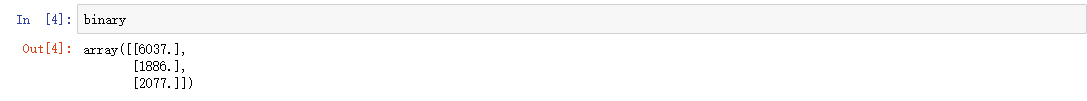# 玩彩票赢钱概率有多少？用Python来告诉你

**游戏规则：**时时彩一种玩法是买尾号。2元一个数字，中奖是20元。每个数字出现的概率相等。

1. 随机购买，人是感性动物，很容易受周围环境干扰。完全随机购买在生活中是不存在的。
2. 分析数字在前面N期出现的概率，选择概率最小的n个数字购买。

 1  import numpy as np 

## 定义玩法一：完全随机购买

  1 2 3 4 5 6 7 8 9 10 11 12 13 14 15 16 17 18 19 20 21 22 23 24 25 26 27 28 29 30 31 32 33 34 35 36 37 38 39 40  def play_lottery(my_money,play_num,money): ''' 随机选择数的中奖率 result：结果字典 num：用于统计每个数字出现次数 my_money：我的余额 play_num：投注期数 money：投注额 flag: 1代表赢钱 0则相反 2表示不输不赢 ''' flag = 1 temp = my_money result = {'0':0, '1':1, '2':2, '3':3, '4':4, '5':5, '6':6, '7':7, '8':8, '9':9} data = np.random.randint(0,10,(play_num,1)) num = np.zeros((10,1)) for i in data: play = np.random.randint(0,10,(5,1)) if i in play: temp = temp + money else: temp = temp - money if result[str(i)] == i: num[i] = num[i] + 1 print('我的余额：',temp) print('开奖结果统计：\n',num,num,num,num,num,num,num,num,num,num) if temp < my_money: flag = 0 elif temp == my_money: flag = 2 return flag 

 1 2 3 4 5  i = 0 binary = np.zeros((3,1)) while i < 10000: binary[play_lottery(1000,10,10)] += 1 i = i + 1可以看出输:赢:平=6037:1886:2077

## 定义玩法二：按照概率购买

  1 2 3 4 5 6 7 8 9 10 11 12 13 14 15 16 17 18 19 20 21 22 23 24 25 26 27 28 29 30 31 32 33 34 35 36 37 38 39 40 41 42 43 44 45  def play_lottery2(my_money,play_num,money): ''' 随机选择数的中奖率 result：结果字典 num：用于统计每个数字出现次数 my_money：我的余额 play_num：投注期数 money：投注额 flag: 1代表赢钱 0则相反 2表示不输不赢 ''' flag = 1 temp = my_money result = {'0':0, '1':1, '2':2, '3':3, '4':4, '5':5, '6':6, '7':7, '8':8, '9':9} data = np.random.randint(0,10,(play_num,1)) num = np.zeros((10,1)) num_first = np.random.randint(0,10,(10,1)) is_one = 1 for i in data: if is_one == 1: play = np.argsort(num_first,axis=0).reshape(-1,1)[0:5] is_one = 0 play = np.argsort(num,axis=0).reshape(-1,1)[0:5] if i in play: temp = temp + money else: temp = temp - money if result[str(i)] == i: num[i] = num[i] + 1 print('我的余额：',temp) print('开奖结果统计：\n',num,num,num,num,num,num,num,num,num,num) if temp < my_money: flag = 0 elif temp == my_money: flag = 2 return flag 

 1 2 3 4 5  i = 0 binary = np.zeros((3,1)) while i < 10000: binary[play_lottery2(1000,10,10)] += 1 i = i + 1